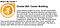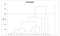# Machine Learning for Humans, Part 3: Unsupervised Learning

## Clustering and dimensionality reduction: k-means clustering, hierarchical clustering, principal component analysis (PCA), singular value decomposition (SVD)

`This series is available as a full-length e-book! Download here. Free for download, contributions appreciated (paypal.me/ml4h)`

How do you find the underlying structure of a dataset? How do you summarize it and group it most usefully? How do you effectively represent data in a compressed format? These are the goals of unsupervised learning, which is called “unsupervised” because you start with unlabeled data (there’s no Y).

The two unsupervised learning tasks we will explore are clustering the data into groups by similarity and reducing dimensionality to compress the data while maintaining its structure and usefulness.

`Examples of where unsupervised learning methods might be useful:- An advertising platform segments the U.S. population into smaller groups with similar demographics and purchasing habits so that advertisers can reach their target market with relevant ads.- Airbnb groups its housing listings into neighborhoods so that users can navigate listings more easily. - A data science team reduces the number of dimensions in a large data set to simplify modeling and reduce file size.`

In contrast to supervised learning, it’s not always easy to come up with metrics for how well an unsupervised learning algorithm is doing. “Performance” is often subjective and domain-specific.

# Clustering

Their white paper reveals that they used centroid clustering and principal component analysis, both of which are techniques covered in this section.

You can imagine how having access to these clusters is extremely useful for advertisers who want to (1) understand their existing customer base and (2) use their ad spend effectively by targeting potential new customers with relevant demographics, interests, and lifestyles.You can actually find out which cluster you personally would belong to by answering a few simple questions in Acxiom’s “What’s My Cluster?” tool.

Let’s walk through a couple of clustering methods to develop intuition for how this task can be performed.

# k-means clustering

The goal of clustering is to create groups of data points such that points in different clusters are dissimilar while points within a cluster are similar.

With k-means clustering, we want to cluster our data points into k groups. A larger k creates smaller groups with more granularity, a lower k means larger groups and less granularity.

The output of the algorithm would be a set of “labels” assigning each data point to one of the k groups. In k-means clustering, the way these groups are defined is by creating a centroid for each group. The centroids are like the heart of the cluster, they “capture” the points closest to them and add them to the cluster.

Think of these as the people who show up at a party and soon become the centers of attention because they’re so magnetic. If there’s just one of them, everyone will gather around; if there are lots, many smaller centers of activity will form.

`Here are the steps to k-means clustering:1. Define the k centroids. Initialize these at random (there are also fancier algorithms for initializing the centroids that end up converging more effectively).2. Find the closest centroid & update cluster assignments. Assign each data point to one of the k clusters. Each data point is assigned to the nearest centroid’s cluster. Here, the measure of “nearness” is a hyperparameter — often Euclidean distance.3. Move the centroids to the center of their clusters. The new position of each centroid is calculated as the average position of all the points in its cluster.Keep repeating steps 2 and 3 until the centroid stop moving a lot at each iteration (i.e., until the algorithm converges).`

That, in short, is how k-means clustering works! Check out this visualization of the algorithm — read it like a comic book. Each point in the plane is colored according the centroid that it is closest to at each moment. You’ll notice that the centroids (the larger blue, red, and green circles) start randomly and then quickly adjust to capture their respective clusters.

Another real-life application of k-means clustering is classifying handwritten digits. Suppose we have images of the digits as a long vector of pixel brightnesses. Let’s say the images are black and white and are 64x64 pixels. Each pixel represents a dimension. So the world these images live in has 64x64=4,096 dimensions. In this 4,096-dimensional world, k-means clustering allows us to group the images that are close together and assume they represent the same digit, which can achieve pretty good results for digit recognition.

# Hierarchical clustering

Hierarchical clustering is similar to regular clustering, except that you’re aiming to build a hierarchy of clusters. This can be useful when you want flexibility in how many clusters you ultimately want. For example, imagine grouping items on an online marketplace like Etsy or Amazon. On the homepage you’d want a few broad categories of items for simple navigation, but as you go into more specific shopping categories you’d want increasing levels of granularity, i.e. more distinct clusters of items.

In terms of outputs from the algorithm, in addition to cluster assignments you also build a nice tree that tells you about the hierarchies between the clusters. You can then pick the number of clusters you want from this tree.

`Here are the steps for hierarchical clustering:1. Start with N clusters, one for each data point.2. Merge the two clusters that are closest to each other. Now you have N-1 clusters.3. Recompute the distances between the clusters. There are several ways to do this (see this tutorial for more details). One of them (called average-linkage clustering) is to consider the distance between two clusters to be the average distance between all their respective members.4. Repeat steps 2 and 3 until you get one cluster of N data points. You get a tree (also known as a dendrogram) like the one below.5. Pick a number of clusters and draw a horizontal line in the dendrogram. For example, if you want k=2 clusters, you should draw a horizontal line around “distance=20000.” You’ll get one cluster with data points 8, 9, 11, 16 and one cluster with the rest of the data points. In general, the number of clusters you get is the number of intersection points of your horizontal line with the vertical lines in the dendrogram.`Source: Solver.com. For more detail on hierarchical clustering, you can check this video out.

# Dimensionality reduction

Dimensionality reduction looks a lot like compression. This is about trying to reduce the complexity of the data while keeping as much of the relevant structure as possible. If you take a simple 128 x 128 x 3 pixels image (length x width x RGB value), that’s 49,152 dimensions of data. If you’re able to reduce the dimensionality of the space in which these images live without destroying too much of the meaningful content in the images, then you’ve done a good job at dimensionality reduction.

We’ll take a look at two common techniques in practice: principal component analysis and singular value decomposition.

# Principal component analysis (PCA)

You’re familiar with the coordinate plane with origin O(0,0) and basis vectors i(1,0) and j(0,1). It turns out you can choose a completely different basis and still have all the math work out. For example, you can keep O as the origin and choose the basis to vectors i’=(2,1) and j’=(1,2). If you have the patience for it, you’ll convince yourself that the point labeled (2,2) in the i’, j’ coordinate system is labeled (6, 6) in the i, j system.

This means we can change the basis of a space. Now imagine much higher-dimensional space. Like, 50K dimensions. You can select a basis for that space, and then select only the 200 most significant vectors of that basis. These basis vectors are called principal components, and the subset you select constitute a new space that is smaller in dimensionality than the original space but maintains as much of the complexity of the data as possible.

To select the most significant principal components, we look at how much of the data’s variance they capture and order them by that metric.

Another way of thinking about this is that PCA remaps the space in which our data exists to make it more compressible. The transformed dimension is smaller than the original dimension.

By making use of the first several dimensions of the remapped space only, we can start gaining an understanding of the dataset’s organization. This is the promise of dimensionality reduction: reduce complexity (dimensionality in this case) while maintaining structure (variance). Here’s a fun paper Samer wrote on using PCA (and diffusion mapping, another technique) to try to make sense of the Wikileaks cable release.

# Singular value decomposition (SVD)

The values in the r*r diagonal matrix Σ are called singular values. What’s cool about them is that these singular values can be used to compress the original matrix. If you drop the smallest 20% of singular values and the associated columns in matrices U and V, you save quite a bit of space and still get a decent representation of the underlying matrix.

To examine what that means more precisely, let’s work with this image of a dog:

We’ll use the code written in Andrew Gibiansky’s post on SVD. First, we show that if we rank the singular values (the values of the matrix Σ) by magnitude, the first 50 singular values contain 85% of the magnitude of the whole matrix Σ.

We can use this fact to discard the next 250 values of sigma (i.e., set them to 0) and just keep a “rank 50” version of the image of the dog. Here, we create a rank 200, 100, 50, 30, 20, 10, and 3 dog. Obviously, the picture is smaller, but let’s agree that the rank 30 dog is still good. Now let’s see how much compression we achieve with this dog. The original image matrix is 305*275 = 83,875 values. The rank 30 dog is 305*30+30+30*275=17,430 — almost 5 times fewer values with very little loss in image quality. The reason for the calculation above is that we also discard the parts of the matrix U and V that get multiplied by zeros when the operation UΣ’V is carried out (where Σ’ is the modified version of Σ that only has the first 30 values in it).

Unsupervised learning is often used to preprocess the data. Usually, that means compressing it in some meaning-preserving way like with PCA or SVD before feeding it to a deep neural net or another supervised learning algorithm.

# Onwards!

Person-in-joke-#1: Y would u ever need to use unsupervised tho?

Person-in-joke-#2: Y? there’s no Y.

# Practice materials & further reading

## On Twitter? So are we. Feel free to keep in touch — Vishal and Samer 🙌🏽

More from Machine Learning for Humans 🤖👶

Written by

Written by

## Vishal Maini

#### Strategy & communications @DeepMindAI. Previously @Upstart, @Yale, @TrueVenturesTEC. Views expressed here are my own.